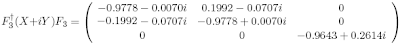occasional meanderings in physics' brave new world

## Friday, October 10, 2008

### CKM Rules III

Using Carl's values for the CKM circulant sum $X + iY$, the Fourier transform gives the sum of a diagonal and codiagonal matrix, which on rearranging rows and columns roughly equalsObserve that the norm of $(-0.9643,0.2614)$ is about 1. This reduces the CKM matrix to even fewer parameters. Note also that the $2 \times 2$ section takes the form

$aI + ib \sigma_{Y} + ic \sigma_{X} + id \sigma_{Z}$

which is a quaternion, close to norm 1. Thus the CKM matrix is expressed as a number $(\alpha, \beta)$, for $\alpha$ a quaternion of norm 1 and $\beta$ a complex number of norm 1. So the transformed matrix is just an element of $SU(2) \times U(1)$. The difference of the $SU(2)$ group factor from an element of the Lie algebra is the value $a = -0.9778$. In fact, the result of the last post shows that this element of the algebra is defined by a transformation

$g = F_{3}^{\dagger} (X + iY) F_{3} + \gamma F_{2}^{\dagger}F_{2}$

where $\gamma$ is a positive real related to $a$.

Aside: Numbers of the form $(\alpha, \beta)$ naturally appear in Connes' noncommutative formulation of the standard model. We expect these parameters to arise from the inclusion of the octonion degrees of freedom.CarlBrannen said...

I'm getting something similar.

Basically, the Fourier transform does a modification on the 3+3i unitary CKM matrix that is like a Koide mass translation.

For that matter, if you look at the structure of the 3+3i numbers, you will see that each triplet has a generation structure. That is, there is a fairly wide gap between consecutive numbers. So it is natural that one would take a Koide conversion.

I want to write a blog post titled "Fourier transforms of (unitary) circulant matrices" and discuss this a little more.

Right now, the way I get the CKM matrix in unitary form is kind of clunky. I should also modify my java program so it searches for the best fit automatically.

And I also have to do something to get an idea of the experimental spread. (But I figured on doing this last after having some sort of a guess on what it should be.)

October 11, 2008 10:01 AMKea said...

I'm too busy waitressing for the next few days to do much. Note that since F2 just cycles the coefficients of the su(2) factor, we have that (F2F3)*(CKM)(F3F2) is a nice SU(2)xU(1) matrix. And since F3F2 is just the MNS matrix, this is obviously a nice type of 'mass to weak' transformation for the quarks.

October 11, 2008 7:09 PMCarlBrannen said...

The thing I'm working on right now is as follows. I'm thinking that the real 1-circ + imag 2-circ is the correct natural form for the CKM and (obviously) the MNS matrices. And taking the Fourier transform is the correct next step.

An MUB set has two different handednesses, right or left. The two contributions to the Fourier transform correspond to these two handedness cases. To get the actual values, you have to integrate over all possible orientations of the MUBs. The total space is 4pi for the first basis vector (which can point anywhere), 2 pi for the second (which can point any perpendicular to the first), and then two discrete possibilities for the third axis, which gives the right and left handed contributions.

For the MNS matrix, all three MUB axes are identical (as they are composed of identical preons). This gives a result which has to be symmetric for the two handedness cases. For the CKM matrix, the preons are of the form 2+1 so it is more restrictive.

Calculations are ongoing, but it is difficult.

October 12, 2008 1:39 PMCarlBrannen said...

Okay, it's the weekend now. I'm going to spend it writing up results and blogging it, or putting it into papers.

Send me an email about your opinion on putting CKM / MNS results into paper on mesons. If I do this, you will have to allow your name on the front.

October 18, 2008 2:23 PM